# How to Use the If Function in Google Sheets

One of the most valuable functions in Google Sheets is the “IF” function.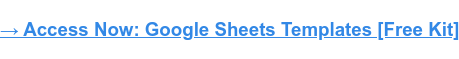Let’s see how it works and see some concrete examples.

## What does the if function do in Google Sheets?

In Google Sheets, the if function allows you to check a condition and return a specific value if it is TRUE.

For example, suppose you have three columns in a spreadsheet: channel, target, and profit. You want to know which channels made more profit than the target.

You can use the IF function to do this – the logic of the formula would look like this: if the target is greater than the profit, it will return “YES”. If not, it will return a “NO”. You can then track this data in another column labeled “Goal Achieved”.

## How to Use the If Function in Google Sheets

When using the if function in Google Sheets, you should use the following syntax:

IF(logical_expression, value_if_true, value_if_false)

Let’s break down what each means:

• Logical expression refers to the condition you are checking in the function.
• Value if true is the value the function will return if the logical expression is true.
• Value if false is the value the function will return if the logical expression is false.

To do this, you will need to use the following logical expression symbols:

• > to designate greater than
• >= to designate equal to or greater than
• < to designate greater than
• <= to designate equal to or less than
• <> to denote not equal
• = to designate equal

Let’s see a concrete example.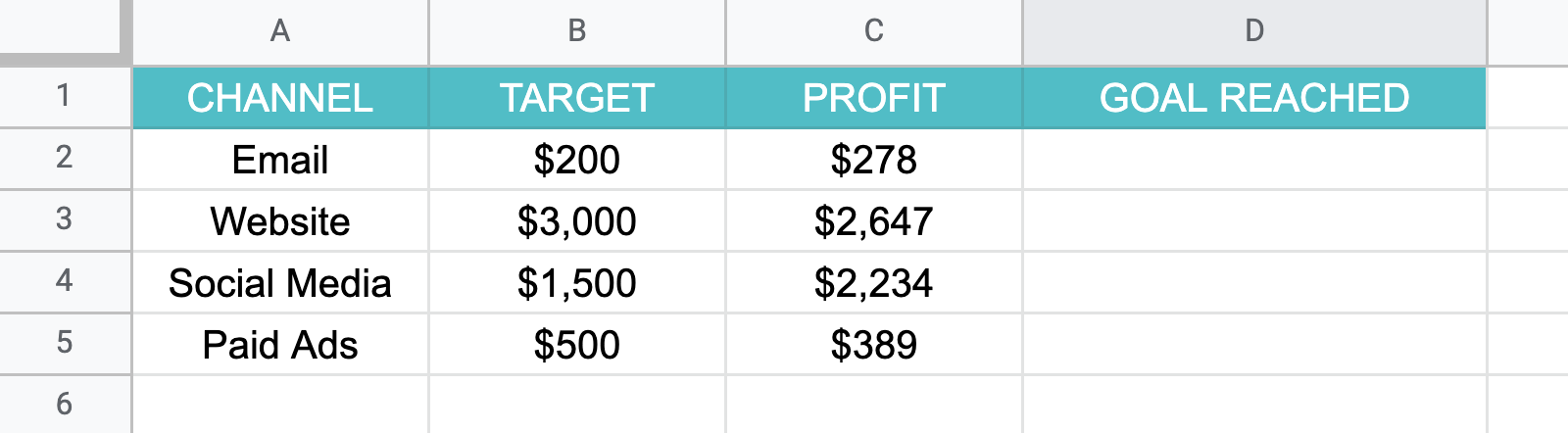Here we have a spreadsheet with performance data for each marketing channel. Each channel had a target revenue next to the actual profit. The last column, “goal met” will be used to quickly respond to channels that have reached the goal.

3. ### Drag the mouse to apply the formula to the relevant cells.

Afraid to forget it? Do not worry. Google Sheets has an auto-fill feature that will show you the formula as you type it.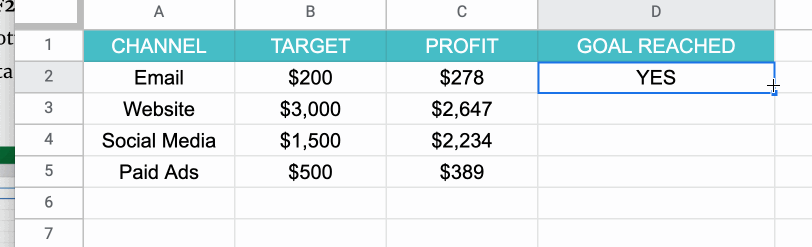In this case, the formula will be: =if (C2 > B2, “YES” , “NO”). To break this down further, the formula says if the messaging channel profit is greater than the target profit, then write “YES”. If it is lower, write “NO”.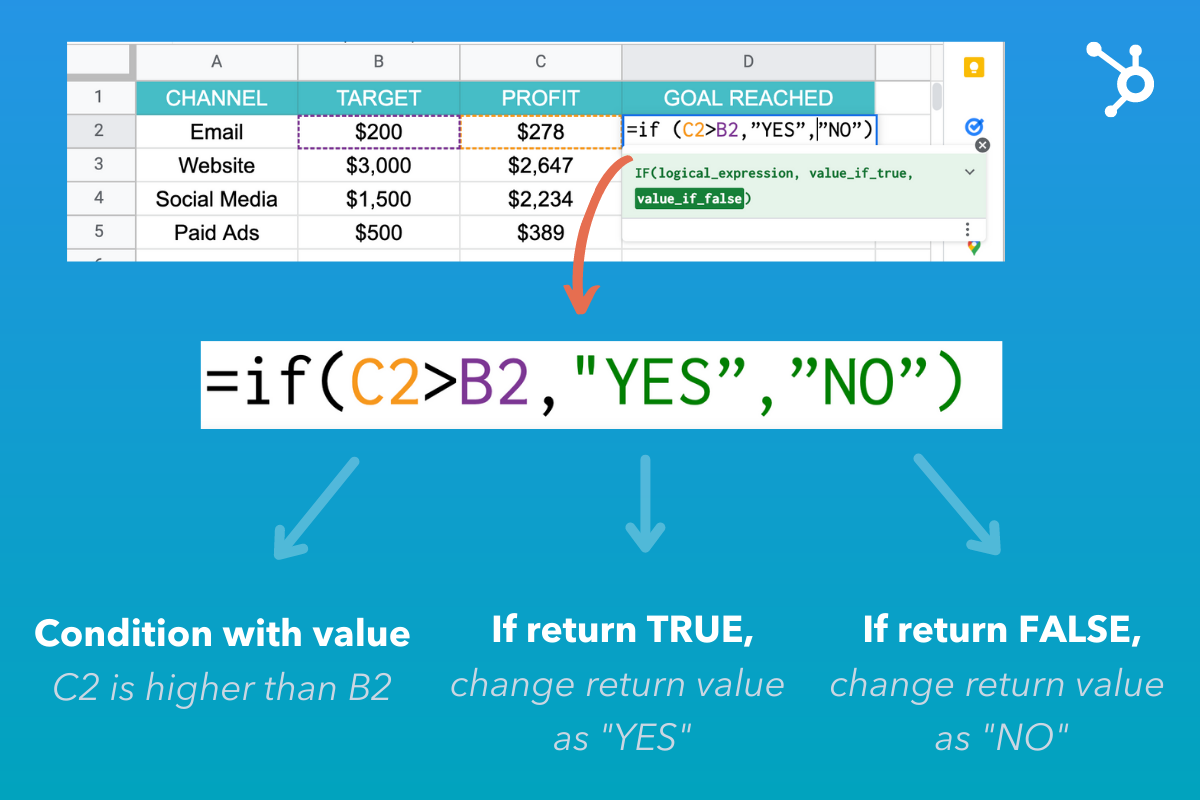Keep in mind that the value if true may be whatever you choose. However, in this example, the simple option was to choose the words “YES” or “NO”.

Since the formula includes words, you must use apostrophes for Google Sheets to read the formula correctly.

## If/Then vs If/And vs If/Or functions

Simply put, with “If-Then” you check that a condition is met. In the example above, it was that one value would be higher than the other. Based on this data, the return value would be “YES” or “NO”.

With “If And” you check several conditions for a “TRUE” value. They must all be “TRUE” for the return value to be “TRUE”.

“If or” is the exact opposite of “If and”. It also checks several conditions, but if any of them is true, it will return a “TRUE” value.

Here is a concrete example:

• If so I’m going to the shop. If you go to the store, the statement is true.
• If and I go to the store and I fill up. You have to go to the store and fill up for the claim to be true. Otherwise, it will be wrong.
• If orI go to the store and I fill up. You can do either task and the statement will be true. The only time it will be wrong if you don’t fill in either.

## How to use the If function with multiple conditions

Suppose you are conducting a survey and you want to easily classify your respondents into two age groups. You could write a formula that indicates that if the respondents are 25 years old or younger, they will be in group “A”. If they are 26 or older, they will be in Group B.

This creates several conditions under which the value can be written. The formula checks the first condition, if it does not apply, it goes to the next one to find the appropriate value.

Let’s see this in action.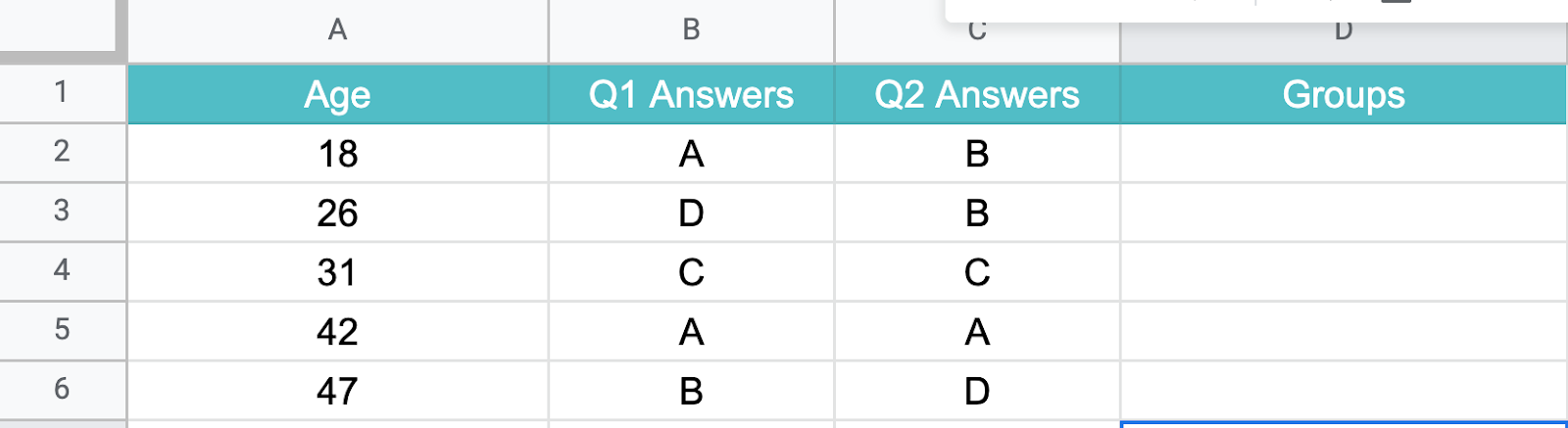Using the example above, your formula would be:

= IF(A2 < = 25, "A", SI(A2 >= 25, “B”))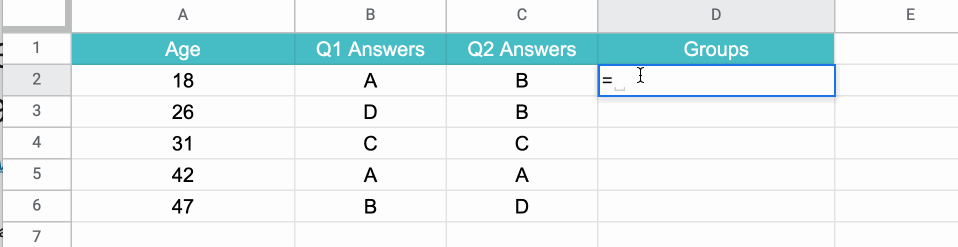## Embedded If function in Google Sheets

In Google Sheets, “nesting” simply refers to placing the “if” function in its own formula to test multiple conditions and return different results based on those tests.

The syntax of the formula is as follows:

=IF(first_statement,value_if_true,IF(second_statement,value_if_true,value_if_false))

It’s that simple.

So here is another feature you can use to speed up your process when using Google Sheets.